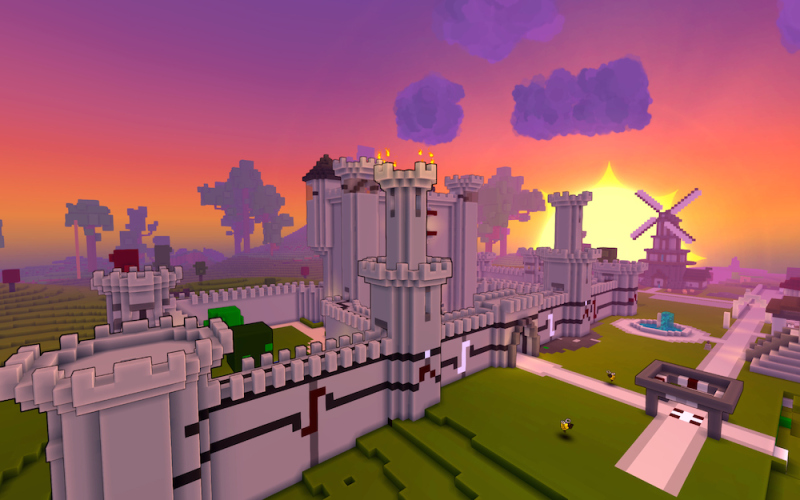## Hihocoder 1291 Buiding in Sandbox

04/07/2016 题解 OJ 校园招聘 Microsoft 微软在线笔试 Hihocoder BFS

## 描述

Little Hi is playing a sandbox voxel game. In the game the whole world is constructed by massive 1x1x1 cubes. The edges of cubes are parallel to the coordinate axes and the coordinates (x, y, z) of the center of each cube are integers.At the beginning there is nothing but plane ground in the world. The ground consists of all the cubes of z=0. Little Hi needs to build everything by placing cubes one by one following the rules:

1. The newly placed cube must be adjacent to the ground or a previously placed cube. Two cubes are adjacent if and only if they share a same face.

2. The newly placed cube must be accessible from outside which means by moving in 6 directions(up, down, left, right, forward, backward) there is a path from a very far place - say (1000, 1000, 1000) in this problem - to this cube without passing through ground or other cubes.

Given a sequence of cubes Little Hi wants to know if he can build the world by placing the cubes in such order.

## 输入

The first line contains the number of test cases T(1 <= T <= 10). For each test case the first line is N the number of cubes in the sequence. The following N lines each contain three integers x, y and z indicating the coordinates of a cube. For 20% of the data, 1 <= N <= 1000, 1 <= x, y, z <= 10. For 100% of the data, 1 <= N <= 100000, 1 <= x, y, z <= 100.

## 输出

For each testcase output “Yes” or “No” indicating if Little Hi can place the cubes in such order.

## 样例提示

In the first test case three cubes are placed on the ground. It’s OK. In the second test case (1, 3, 2) is neither on the ground nor adjacent to previous cubes. So it can’t be placed. In the last test case (2, 2, 1) can not be reached from outside. So it can’t be placed.

3
3
1 1 1
1 2 1
1 3 1
3
1 1 1
1 2 1
1 3 2
17
1 1 1
1 2 1
1 3 1
2 3 1
3 3 1
3 2 1
3 1 1
2 1 1
2 1 2
1 1 2
1 2 2
1 3 2
2 3 2
3 3 2
3 2 2
2 2 2
2 2 1


Yes
No
No


BFS

## 代码

#include <stdio.h>
#include <string.h>
#include <algorithm>
#include <queue>

using std::max;
using std::queue;
const int MAXN = 105;
int cube[MAXN][MAXN][MAXN];
int data[100000 + 5];

const int dx[] = { 0, 0, 1,-1, 0, 0};
const int dy[] = { 0, 0, 0, 0, 1,-1};
const int dz[] = { 1,-1, 0, 0, 0, 0};
bool bfs(int x,int y,int z,int lim, int tag);

struct State{
int x,y,z;
State():x(0),y(0),z(0){}
State(int a,int b,int c):x(a),y(b),z(c){}
};

{
int a,b,c;
if(z == 1) return true;
for(int i = 0; i < 6; i++)
{
a = x + dx[i];
b = y + dy[i];
c = z + dz[i];
if( a > 0 && b > 0 && c > 0 && cube[a][b][c])
return true;
}
return false;
}

int main()
{
int t,x,y,z,lim,cnt;
bool flag;
scanf("%d",&t);
while(t--)
{
lim  = 0;
flag = true;
scanf("%d",&cnt);
memset(cube,0,sizeof(cube));
for(int i = 0; i < cnt; i++)
{
scanf("%d%d%d",&x,&y,&z);
flag = false;
cube[x][y][z] = (i + 1);
data[i] = x;
data[i] = y;
data[i] = z;
lim = max(max(lim,x),max(y,z));
}
lim += 1;
if(flag)
{
bfs(lim,lim,lim,lim,cnt+5);
while(cnt-- && flag)
flag = bfs(data[cnt],data[cnt],data[cnt],lim,cnt + 5);
}
if(flag)
puts("Yes");
else
puts("No");
}
return 0;
}

bool bfs(int x,int y,int z,int lim,int tag)
{
queue<State>  que;
que.push(State(x,y,z));
cube[x][y][z] = tag;
bool ret = false;
State now;
int a,b,c;
while(!que.empty())
{
now = que.front(); que.pop();
for(int i = 0; i < 6; i++)
{
a = now.x + dx[i];
b = now.y + dy[i];
c = now.z + dz[i];
if( a < 0 || b < 0 || c < 1 || a > lim || b > lim || c > lim)
continue;
if(cube[a][b][c] > tag)
ret = true;
if(cube[a][b][c])
continue;
cube[a][b][c] = tag;
que.push(State(a,b,c));
}
}
return ret;
}

/**
3
1 1 1
1 2 1
1 3 2
17
1 1 1
1 2 1
1 3 1
2 3 1
3 3 1
3 2 1
3 1 1
2 1 1

2 1 2
1 1 2
1 2 2
1 3 2
2 3 2
3 3 2
3 2 2
2 2 2
2 2 1

**/